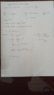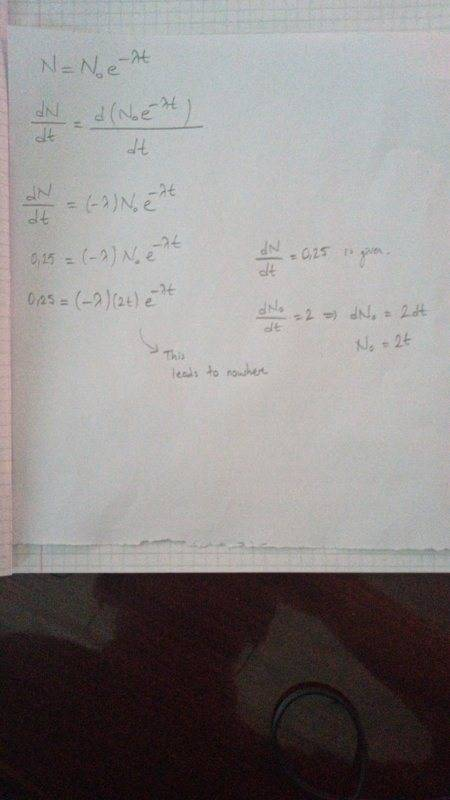# I did not understand this derivative -- help please

cemtu
Homework Statement:
Mathematical Methods in Physics
Relevant Equations:
Derivative
I have no idea how this derivative was taken.

#### Attachments

•derivative-full question.jpg
30.8 KB · Views: 158

It's wrong. ##N_{0}## is a constant (assuming the conventional interpretation of ##N_0## as the value of ##N## at ##t=0##). ##\frac{dN_0}{dt}## is always going to be ##0## and doesn't really make any sense. And to go one further, you still need to derive ##e^{-\lambda t}## wrt ##t##.

cemtu
dN0/dt = 2 is given thus N0 is 2t. There is no problem there sir.

Homework Helper
Gold Member
2022 Award
dN0/dt = 2 is given thus N0 is 2t. There is no problem there sir.
Your original post makes no sense. If you want any help you'll have to organise it into something meaningful.

•etotheipi
cemtu
Okay right away.

cemtu
Your original post makes no sense. If you want any help you'll have to organise it into something meaningful.
I put the whole question and its solution.

Are you sure you don't mean ##A_{0} = \left[-\frac{dN}{dt} \right]_{t=0} = 2##?

Of course, start off with the equation you wrote of ##N = N_{0}e^{-\lambda t}##. Then you can derive both sides with respect to ##t## to obtain a relation pertaining to the activities.

How would you derive the RHS wrt ##t##? Or perhaps more specifically, what can we do about the constant out the front?

cemtu
Are you sure you don't mean ##A_{0} = \left[-\frac{dN}{dt} \right]_{t=0} = 2##?

Of course, start off with the equation you wrote of ##N = N_{0}e^{-\lambda t}##. Then you can derive both sides with respect to ##t## to obtain a relation pertaining to the activities.

How would you derive the RHS wrt ##t##? Or perhaps more specifically, what can we do about the constant out the front?
I know how to derive however our professor just replaced N with dN/dt (LHS) and N0 with dN0/dt (RHS). I don't know how. He didnt took the derivative as the way you and I want.

I know how to derive however our professor just replaced N with dN/dt (LHS) and N0 with dN0/dt (RHS). I don't know how. He didnt took the derivative as the way you and I want.

I can't speak for what your professor was trying to imply however I can say that ##\frac{dN_{0}}{dt}## is zero, as is the case for all constants. It's essentially a meaningless statement.

What do you get if you derive both sides of ##N = N_0 e^{-\lambda t}## with respect to ##t##?

•cemtu
I can't speak for what your professor was trying to imply however I can say that ##\frac{dN_{0}}{dt}## is zero, as is the case for all constants. It's essentially a meaningless statement.

What do you get if you derive both sides of ##N = N_0 e^{-\lambda t}## with respect to ##t##?
HereLast edited by a moderator:
Homework Helper
Gold Member
2022 Award
Here
I suspect this might be an initial value problem. But, you've misunderstood what's been given as initial values.

Without seeing the problem stated clearly, we are all just guessing.

•etotheipi
Here

You've done the differentiation right, ##\frac{dN}{dt} = -\lambda N_{0} e^{-\lambda t} \implies A = A_{0}e^{-\lambda t}## since ##A = \lambda N##.

You must stop using ##\frac{dN_{0}}{dt}##. It is wrong. I strongly suspect the intended meaning is the rate of change of ##N## at ##t=0##, however we might just denote this (negative) ##A_{0}## (or that more clunky expression in post #7).

You should be able to just plug in the ratio of the activities.

•cemtu
cemtu
You've done the differentiation right, ##\frac{dN}{dt} = -\lambda N_{0} e^{-\lambda t} \implies A = A_{0}e^{-\lambda t}## since ##A = \lambda N##.

You must stop using ##\frac{dN_{0}}{dt}##. It is wrong. I strongly suspect the intended meaning is the rate of change of ##N## at ##t=0##, however we might just denote this (negative) ##A_{0}## (or that more clunky expression in post #7).

You should be able to just plug in the ratio of the activities.
Sir, thank you.

Sir, thank you.

You're welcome, though no need to call me 'sir', I haven't met the Queen yet!

••